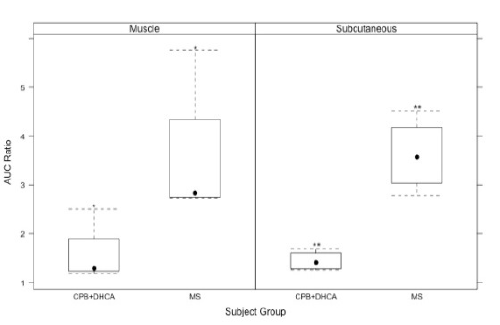Figure 3: Box and whisker plots of median AUC ratios. Muscle AUC/total plasma AUC ratios for piglets that underwent CPB +DHCA (n=4) were significantly decreased compared to MS only (n=4) (*P=0.03). Subcutaneous AUC/total plasma AUC ratios for piglets that underwent CPB+DHCA (n=4) were significantly decreased compared to MS only (n=4) (**P=0.03).